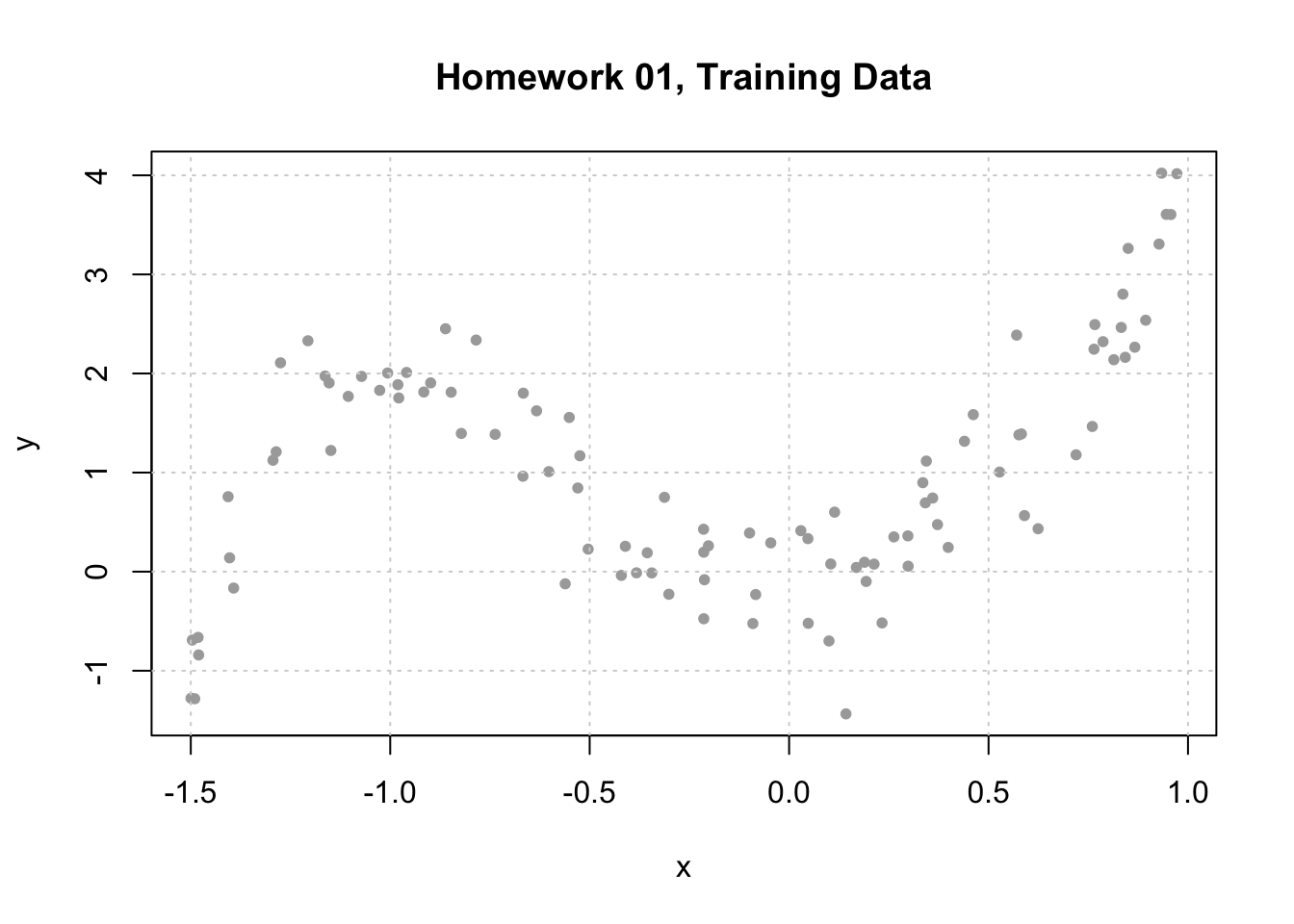Please see the homework policy document for detailed instructions and some grading notes. Failure to follow instructions will result in point reductions.

“The fool wonders, the wise man asks.”

Benjamin Disraeli

This homework will use data in hw01-trn-data.csv and hw01-tst-data.csv which are train and test datasets respectively. Both datasets contain a single predictor x and a numeric response y. The following chunk imports this data.

hw01_trn_data = read.csv("hw01-trn-data.csv")
hw01_tst_data = read.csv("hw01-tst-data.csv")

For this assignment, you may only use the following packages:

library(FNN)
library(rpart)
library(knitr)
library(kableExtra)

## Exercise 1 (Polynomial Models)

Fit a total of five polynomial models to the training data that can be used to predict y from x. Use polynomial degrees of 1, 3, 5, 7, and 9. For each, calculate both train and test RMSE. Do not output these results directly, instead summarize the results with a single well labeled plot that shows both train and test RMSE as a function of the degree of the polynomial fit.

## Exercise 2 (KNN Models)

Fit a total of five KNN models to the training data that can be used to predict y from x. Use k (number of neighbors) values of 1, 11, 21, 31, and 41. For each, calculate both train and test RMSE. Do not output these results directly, instead summarize the results with using a well-formatted markdown table that shows k, train RMSE and test RMSE.

## Exercise 3 (Tree Models)

Fit a total of five tree models to the training data that can be used to predict y from x. To do so, use the rpart() function from the rpart package. The rpart() syntax is very similar to lm(). For example:

rpart(y ~ x, data = some_data, control = rpart.control(cp = 0.5, minsplit = 2))

This code fits a tree with a cost complexity parameter of 0.5, as defined using the cp argument to rpart.control. We will consider this to be the single tuning parameter of tree fitting. (More on this much later in the course.) The minsplit argument could also be considered a tuning parameter, but we will keep it fixed at 2.

Use cp values of 0, 0.001, 0.01, 0.1, and 1. For each, calculate both train and test RMSE. Do not output these results directly, instead summarize the results with using a well-formatted markdown table that shows cp, train RMSE and test RMSE.

## Exercise 4 (Visualizing Results)

Add lines (curves) to the following plot which correspond to the fitted model for the best polynomial model, best KNN model, and best tree model based on the results of the previous exercises. Use different line types and colors for the different models. Add a legend to indicate which line is which model.

plot(y ~ x, data = hw01_trn_data, col = "darkgrey", pch = 20,
main = "Homework 01, Training Data")
grid()## Exercise 5 (Concept Checks)

(a) Which, if any, of the polynomial models are likely underfitting based on the results you obtained?

(b) Which, if any, of the polynomial models are likely overfitting based on the results you obtained?

(c) Which, if any, of the KNN models are likely underfitting based on the results you obtained?

(d) Which, if any, of the KNN models are likely overfitting based on the results you obtained?

(e) Which, if any, of the tree models are likely underfitting based on the results you obtained?

(f) Which, if any, of the tree models are likely overfitting based on the results you obtained?